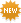Let The Day Begin lyrics by Samhain - original song full text. Official Let The Day Begin lyrics, 2020 version | LyricsMode.comRequest & respond explanations
• Don't understand the meaning of the song?
• Highlight lyrics and request an explanation.
• Click on highlighted lyrics to explain.
Samhain – Let The Day Begin lyrics
Let the day begin
Let the horror start
Let the fury swell
Let the skies all cry
Let the day begin

Let the fun begin
Let the worlds collide
Let the day begin
Let humanity spin
Let the day begin

Inherent time is at hand
So then let the day begin
So then let the day begin

Let the world stand bare
Let it stand on trial
Let it open up
Let the tides explode
Let the day begin

Let the Jews all scream
Let the Catholics burn
Let the Protestants hide
Let the false ones wallow in their own demise

Let the fun begin
Let the worlds collide
Let the day begin
Let humanity spin
Let the day begin

Inherent time is at hand
So then let the day begin
So then let the day begin

Inherent time is at hand
So then let the day begin
So then let the day begin

Inherent time is at hand
So then let the day begin
So then let the day begin

And the earth stands stripped
And its imminent rape
Let them get their due
Let them feel the pain
That they gave to you

Let the day begin
Let the horror start
Let the fury swell
Let the skies all cry
Let the day begin

Inherent time is at hand
So then let the day begin
So then let the day begin

Inherent time is at hand
So then let the day begin
So then let the day begin
×

Lyrics taken from /lyrics/s/samhain/let_the_day_begin.html

• Email
• Correct

## Let The Day Beginmeanings

Know what this song is about? Does it mean anything special hidden between the lines to you? Share your meaning with community, make it interesting and valuable. Make sure you've read our simple .
U
Min 50 words
Good
Awesome!

U
Min 50 words